## You are reacting hydrogen gas with tin oxide to purify tin. SnO2 + 2 H2 → Sn + 2 H2O. You have 45.8L of H2 gas and 351.3g of SnO2. What will

Question

You are reacting hydrogen gas with tin oxide to purify tin. SnO2 + 2 H2 → Sn + 2 H2O. You have 45.8L of H2 gas and 351.3g of SnO2. What will be the limiting reactant, and how much of the excess reactant will be left over?

in progress 0
6 months 2021-07-22T15:08:18+00:00 1 Answers 42 views 0

## Answers ( )

1. Answer: The limiting reactant is hydrogen gas and the mass of excess reactant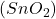left over is 197.43 g

Explanation:

Limiting reagent is defined as the reagent which is completely consumed in the reaction and limits the formation of the product.

Excess reagent is defined as the reagent which is left behind after the completion of the reaction.

The number of moles is defined as the ratio of the mass of a substance to its molar mass.

The equation used is:

……(1)

We are given:

Given mass of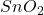= 351.3 g

Molar mass of= 150.71 g/mol

Putting values in equation 1, we get: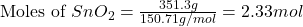At STP conditions:

22.4 L of volume is occupied by 1 mole of a gas

Applying unitary method:

45.8 L of volume will be occupied by =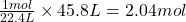of hydrogen gas

For the given chemical reaction: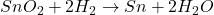By stoichiometry of the reaction:

If 2 moles of hydrogen gas reacts with 1 mole ofSo, 2.04 moles of hydrogen gas will react with =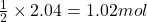ofAs the given amount ofis more than the required amount. Thus, it is present in excess and is considered as an excess reagent.

Thus, hydrogen gas is considered a limiting reagent because it limits the formation of the product.

Moles of excess reactant () left = [2.33 – 1.02] = 1.31 moles

We know, molar mass of= 150.71 g/mol

Putting values in equation 1, we get: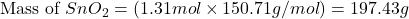Hence, the limiting reactant is hydrogen gas and the mass of excess reactantleft over is 197.43 g